Next: Combination of SAMM with Up: The FAMUSAMM algorithm Previous: Structure Adapted Multipole Method

## Multiple Time Step Methods

Another class of approximation schemes, the multiple-time-step methods [28, 29, 32, 16, 33] , exploit complementary properties of protein electrostatics for the same purpose; they take advantage of temporal regularities. The so-called distance class methods, in particular, are based on the observation that forces originating from distant atoms fluctuate more slowly than forces from atoms nearby (see Figure 3). The slowly fluctuating forces may be evaluated less frequently than the fast ones and may be extrapolated at the time steps in between. Such extrapolation is required as the numerical integration of the dynamical equations needs all forces at every integration time step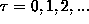, which discretizes the simulation time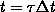, where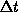is the integration time step size.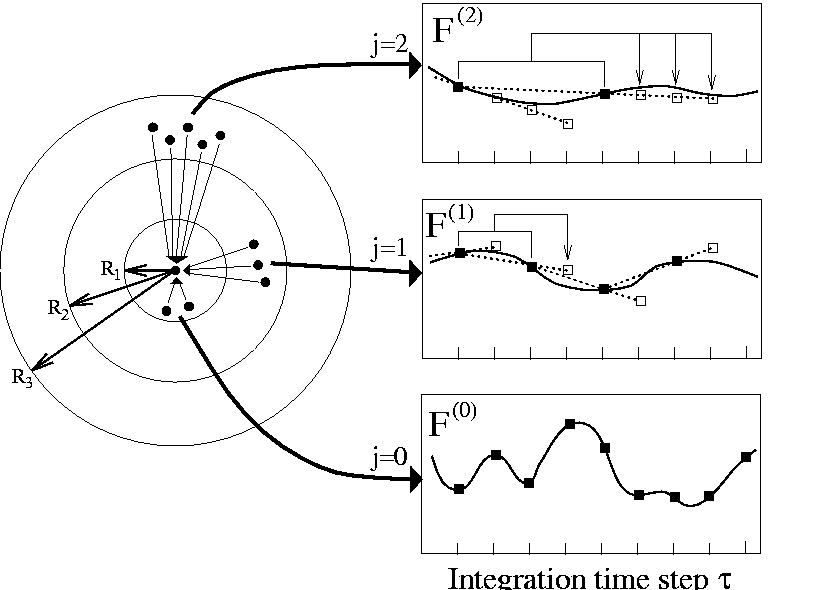Figure: (left) Distance classes j=0,1,2,.., are defined for an atom (central dot) by a set of radii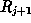generating a series of spherical shells; (right) also shown is for each distance class the temporal evolution of the total force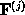acting on the selected atom originating from all atoms in the respective distance class; along with the exact forces (solid line) and their exact values (filled squares) also linear force extrapolations (dotted lines) and resulting force estimates (empty squares) are depicted.

The left part of Figure 3 illustrates how distance classes j can be defined by a set of increasing radii. The set of atoms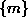at positions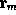satisfying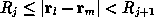makes up the distance class j of particle l at position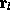. As is also indicated in Figure 3 (right part), for each particle l the sum of forcesarising from particles in distance class j is calculated explicitly every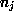-th integration time step (filled squares). Each of these time steps is called a macro integration step. A common choice  is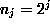. Correspondingly, the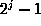elementary time steps within the cycle of a macro integration step are called micro integration steps. As sketched in Figure 3, at each stepis estimated from two forces calculated at previous macro integration steps by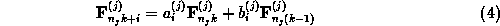using appropriate extrapolation coefficients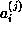and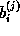. The lower index of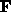denotes the absolute integration time step number and is expressed in terms of the macro integration step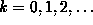and the cyclic micro integration step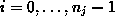;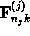and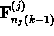are explicitly calculated at the macro integration steps k and k-1.

The hierarchical extrapolation procedure sketched above is capable to save an enormous amount of computer time as it frequently avoids the most time consuming step, i.e., the exact evaluation of all interactions. Here computational speed is gained at the cost of an increased demand for memory: for each atom and each distance class two previous forces have to be kept in memory.

Various choices for the extrapolation coefficients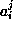and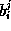have been discussed [16, 33, 37]; both the linear extrapolation (illustrated in Figure 3) and the so-called DC-1d algorithm have been found promising . Although the linear extrapolation defined by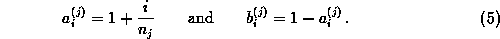entails smaller discontinuities for the extrapolated forces than the DC-1d algorithm, it leads to a larger energy transfer into a simulation system by algorithmic noise . The DC-1d scheme employs the coefficients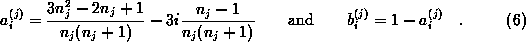It is a priori not clear, whether the quoted properties of these extrapolation schemes will pertain, if they are combined with the SAMM procedure into our new FAMUSAMM scheme, which will be explained in the next section. Therefore, using test simulations we will subsequently check both extrapolation methods for their suitability within our combination method.Next: Combination of SAMM with Up: The FAMUSAMM algorithm Previous: Structure Adapted Multipole Method

Helmut Grubmueller
Wed Apr 30 15:40:09 MET DST 1997